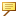minimum_angle_dimension
If the angle between the references is less than the value defined in the detail option, the references are assumed to be parallel. When you create a driven dimension annotation, a linear dimension is created between the references. For example, if the value of the angular value between the references is 3 and the value of the detail option is 4, a linear dimension is created between the references.
If the angle between the references is more than the value defined in the detail option, an angular dimension is created between the references. For example, if the value of the angular value between the references is 5 and the value of the detail option is 4, an angular dimension is created between the references.If you open a model created in Creo Parametric 4.0 M070 and earlier, the Detail option value is set to the current value of the configuration option minimum_angle_dimension.